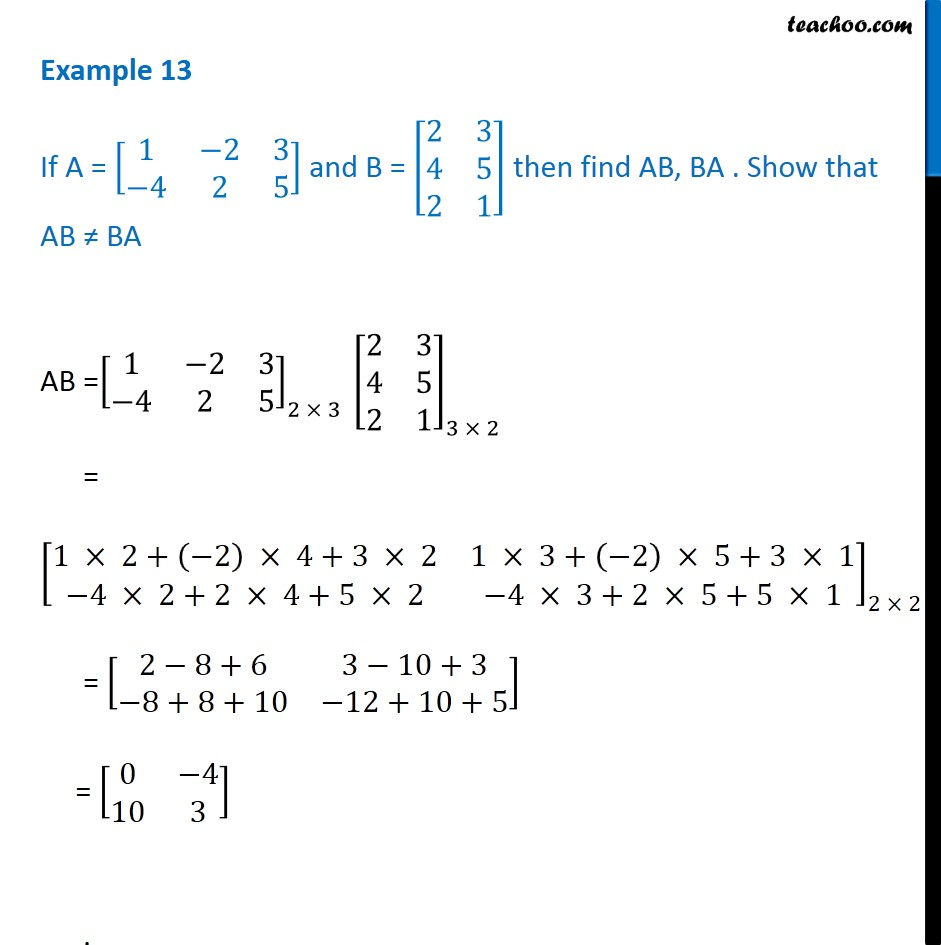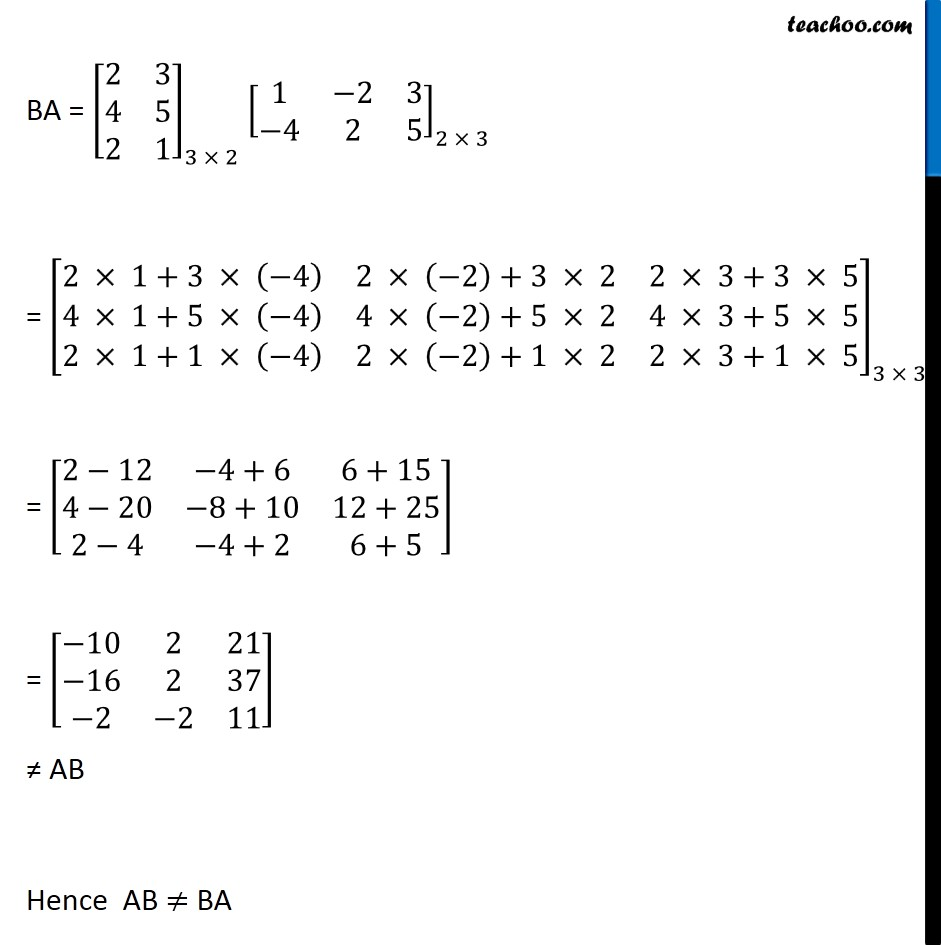Multiplication of matrices

Chapter 3 Class 12 Matrices
Concept wiseLearn in your speed, with individual attention - Teachoo Maths 1-on-1 Class

### Transcript

Example 13 If A = [■8(1&−2&3@−4&2&5)] and B = [■8(2&[email protected]&[email protected]&1)] then find AB, BA . Show that AB ≠ BA AB =[■8(1&−2&3@−4&2&5)]_(2 × 3 ) [■8(2&[email protected]&[email protected]&1)]_(3 × 2) = [■8(1 × 2+(−2) × 4+3 × 2&1 × 3+(−2) × 5+3 × 1@−4 × 2+2 × 4+5 × 2&−4 × 3+2 × 5+5 × 1)]_(2 × 2 ) = [■8(2−8+6&3−10+3@−8+8+10&−12+10+5)] = [■8(0&−[email protected]&3)] . BA = [■8(2&[email protected]&[email protected]&1)]_(3 × 2) [■8(1&−2&3@−4&2&5)]_(2 × 3) = [■8(2 × 1+3 × (−4)&2 × (−2)+3 × 2&2 × 3+3 × [email protected] × 1+5 × (−4)&4 × (−2)+5 × 2&4 × 3+5 × [email protected] × 1+1 × (−4)&2 × (−2)+1 × 2&2 × 3+1 × 5)]_(3 × 3 ) = [■8(2−12&−4+6&[email protected]−20&−8+10&[email protected]−4&−4+2&6+5)] = [■8(−10&2&21@−16&2&37@−2&−2&11)] ≠ AB Hence AB ≠ BA